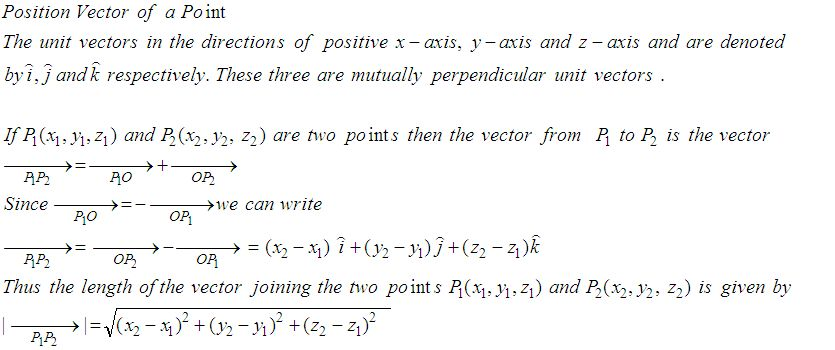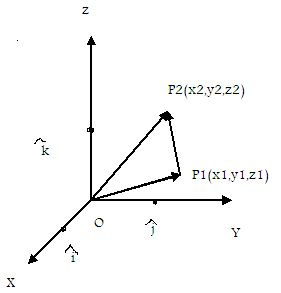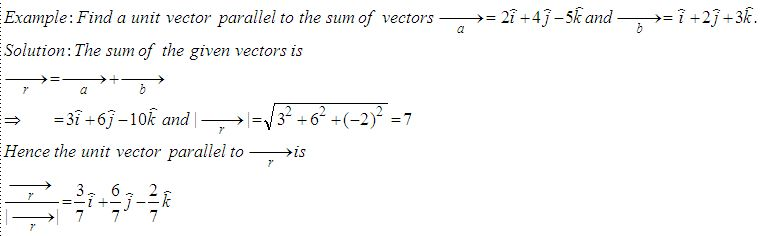Email us to get an instant 20% discount on highly effective K-12 Math & English kwizNET Programs!

Online Quiz (WorksheetABCD)

Questions Per Quiz = 2 4 6 8 10

High School Mathematics - 25.9 Position Vector of a PointDirections: Answer the following.
 Q 1: If P = 2,4,7 and P2 = (-4, -1, 5) then find vector P1P2.6i+5j+3k-6i-5j-2k-6i+5j Q 2: Find the unit vector in the direction of P(1,2,3) towards Q(4,5,6).(i+j+k)/Ö38j-2k6i+5j+2k Q 3: Find the vector with initial point P(6,-2) and terminal point Q(4,-8).-2i+10j6i-9j8i+2j Q 4: Find the vector in the direction of the vector -i+2j+2k that has magnitude 7.7(-i+2j+2k)/3i-j+k-3i+2j Q 5: Find the unit vector in the direction of of P(1,2) and Q(4,5).(i+j)/Ö22i+5j3i-2j Q 6: Find a unit vector in the direction from P(3,2) towards Q(5,6).i/Ö5 + 2j/Ö52i+34i+5 Q 7: Find a unit vector parallel to the sum of vectors a = 2i+4j-5k, b = i+2j+3k3/7i + 6/7j - 2/7 k2-i88i+4j-3k Q 8: Find the vector joining the initial point P(-4,2) and terminal point Q(0,-4) 4i-6j4i+6j8i-6j Question 9: This question is available to subscribers only! Question 10: This question is available to subscribers only!